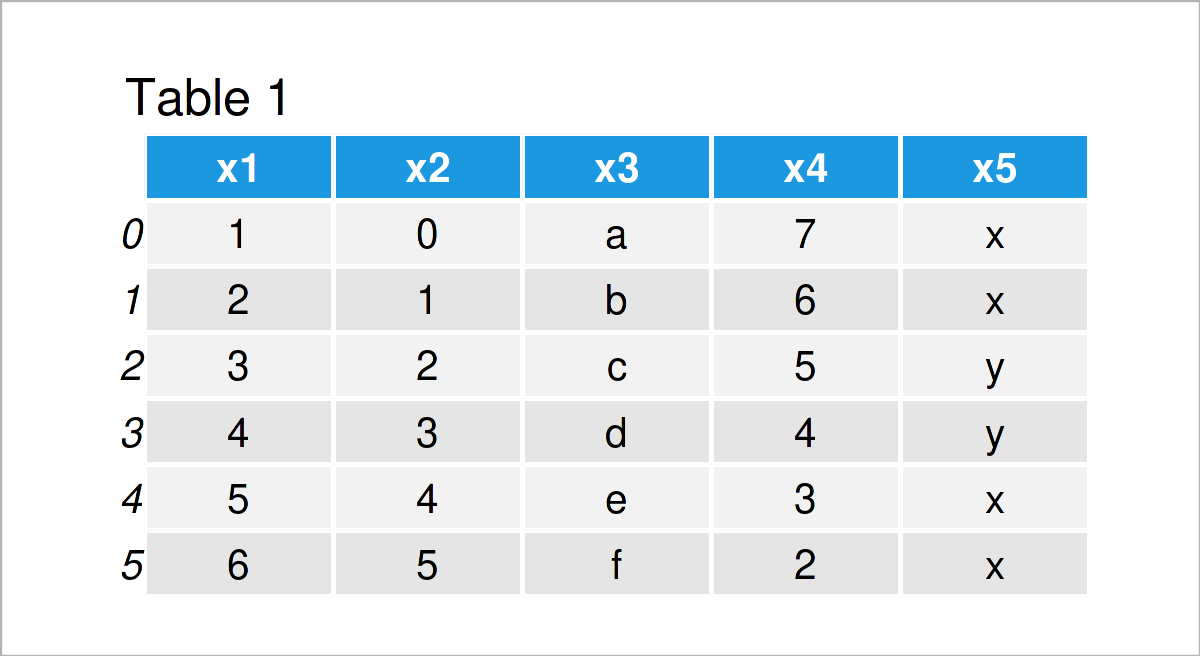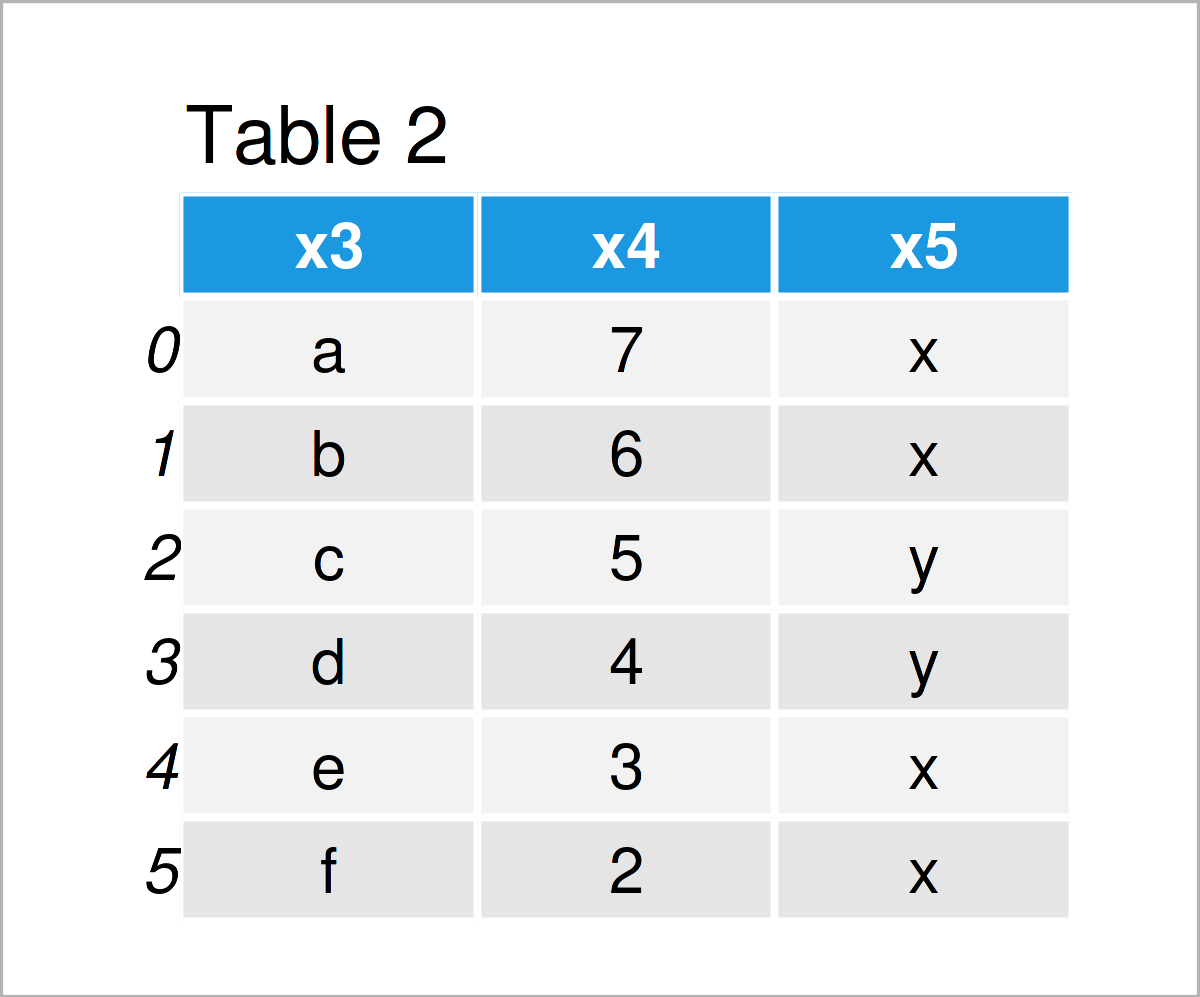# Drop First & Last N Columns from pandas DataFrame in Python (2 Examples)

In this article, I’ll illustrate how to remove the first and last N variables from a pandas DataFrame in Python.

Let’s dive into it:

## Exemplifying Data & Libraries

In order to use the functions of the pandas library, we first have to load pandas:

`import pandas as pd                       # Load pandas library`

Furthermore, have a look at the following example pandas DataFrame:

```data = pd.DataFrame({'x1':range(1, 7),    # Create pandas DataFrame
'x2':range(0, 6),
'x3':['a', 'b', 'c', 'd', 'e', 'f'],
'x4':range(7, 1, - 1),
'x5':['x', 'x', 'y', 'y', 'x', 'x']})
print(data)                               # Print pandas DataFrame```As you can see based on Table 1, our example data is a DataFrame containing six rows and five columns.

## Example 1: Delete First N Columns from pandas DataFrame

This example demonstrates how to drop the first N columns from a pandas DataFrame in Python.

For this task, we can use the iloc attribute as shown below:

```data_drop_first = data.iloc[:, 2:]        # Remove first columns
print(data_drop_first)                    # Print last columns```As shown in Table 2, we have created a pandas DataFrame subset where the first two variables x1 and x2 have been excluded.

## Example 2: Delete Last N Columns from pandas DataFrame

The following Python syntax illustrates how to remove the last N columns from a pandas DataFrame in Python.

To achieve this, we can use the iloc attribute once again. Consider the Python syntax below:

```data_drop_last = data.iloc[:, :3]         # Remove last columns
print(data_drop_last)                     # Print first columns```As shown in Table 3, the previous Python syntax has created another pandas data set. In this case, the last two variables have been deleted.

## Video & Further Resources

If you need further explanations on the Python syntax of this article, I recommend watching the following video on my YouTube channel. I’m explaining the Python programming syntax of this article in the video:

Please accept YouTube cookies to play this video. By accepting you will be accessing content from YouTube, a service provided by an external third party.If you accept this notice, your choice will be saved and the page will refresh.

In addition, you may want to have a look at the related tutorials on Statistics Globe. Some tutorials that are related to the removal of the first and last N variables from a pandas DataFrame are shown below.

You have learned in this tutorial how to delete the first and last N columns from a pandas DataFrame in the Python programming language. Don’t hesitate to let me know in the comments below, if you have further questions.

Subscribe to the Statistics Globe Newsletter